# R语言—-逻辑回归实例（R包mtcars数据集举例）

https://blog.csdn.net/weixin_41744624/article/details/105506951

# 异常值检验

``````boxplot(mtcars\$mpg~am,mtcars)
``````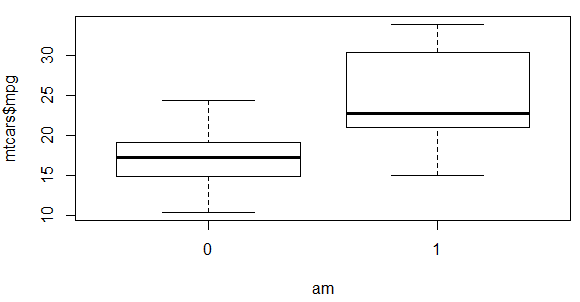``````rowSums(is.na(raw_data))
``````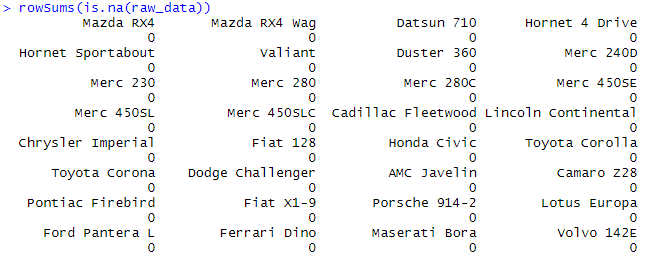``````>d_n <- raw_data[which(rowSums(is.na(raw_data)) > 0),]
>d_nn <- raw_data[-which(rowSums(is.na(raw_data)) > 0),]
``````

# 提取数据，分割训练集，测试集

``````d <- raw_data
# 保证实验可重复性
set.seed(110)
train <- createDataPartition(y=d\$am,p=0.8,list=FALSE)

d_train <- d[train,]
d_test <- d[-train,]
``````

# 回归模型建立

``````glm <- glm(am~.,family = binomial(link ='logit'),data = d_train)
``````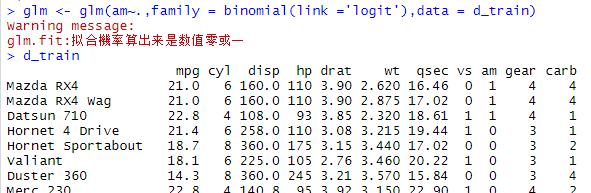https://www.cnblogs.com/runner-ljt/p/4574275.html

https://blog.csdn.net/weixin_41744624/article/details/105725381

## 逐步回归分析

``````logit.step <- step(glm, direction = "both")
``````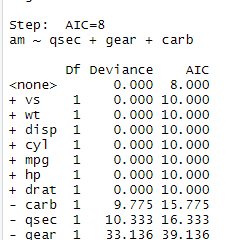``````drop1(logit.step)
``````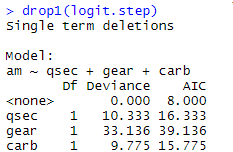## 新模型建立及预测结果

``````# 使用去除变量后的数据进行模型训练
glm <- glm(am ~ qsec+carb,family = binomial(link ='logit'),data = d_train)
#预测模型
pre_test=predict(glm,newdata=d_test,type="response")
#返回预测模型和原数据的混淆矩阵，查看预测结果
confusionMatrix(as.factor(num_test),as.factor(d_test\$am))
``````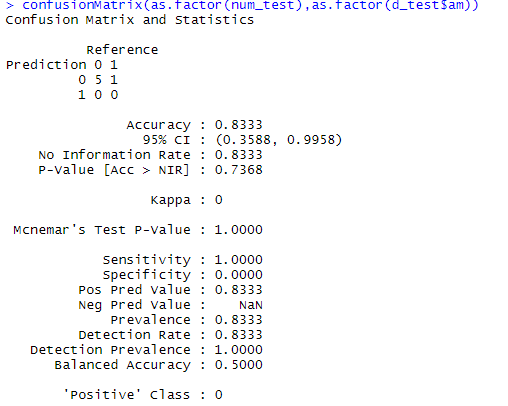THE END